Next: About this document ... Up: lab_template Previous: lab_template

Subsections

# Uniqueness of the Taylor series

## Purpose

The purpose of this lab is to acquaint you with several useful techniques for generating Taylor series. These techniques include substitution and multiplication and division of series by simple polynomials.

## Getting Started

To assist you, there is a worksheet associated with this lab that contains examples and even solutions to some of the exercises. You can copy that worksheet to your home directory with the following command, which must be run in a terminal window, not in Maple.

cp ~bfarr/Tayseries_start.mws ~


You can copy the worksheet now, but you should read through the lab before you load it into Maple. Once you have read to the exercises, start up Maple, load the worksheet Tayseries_start.mws, and go through it carefully. Then you can start working on the exercises.

## Background

The general form of the Taylor series representation with base pointof a function
is given by the following

Definition 1   Suppose thatis a smooth function in some open interval containingand that for every fixed value of
in this interval, it can be shown that the Taylor polynomial remainder
goes to zero as. Then the Taylor series representation with base pointof the functionis given by
In class we will derive the following four important Taylor series,
where the last series is really the formula for the sum of a geometric series in disguise.

Once you have a Taylor series representation for a function, it can be used in several ways to generate Taylor series representations of related functions. This is because of the following theorem.

Theorem 1   Suppose
satisfies
for allin some interval around
. Then,Thus a function cannot have more than one power series in
that represents it.

The rest of the Background describes several different techniques for generating Taylor series of functions that are related to Taylor series that are already known. The four techniques are substitution, multiplication and division, integration, and differentiation. We have already seen examples of integration and differentiation with Taylor polynomials, but we haven't talked about the first two techniques yet.

### Substitution

To get a Taylor series for
, you could go through the standard procedure of differentiating and substituting into the general formula, but an easier (and also correct) procedure is to take the series forand substitute
for, obtainingWriting out the first few terms givesBy the uniqueness theorem, this must be the Taylor series for.

The most commonly useful Taylor series have base point
and that is what we will focus on in this lab. The technique of substitution is most useful if the substitution is of the form
whereis a constant and
is a positive integer. For example, the series for
is easy to obtain as
but it is not clear at all if the following substitution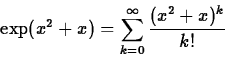produces a useful result. This is because you would have to do a lot of work expanding powers of
and collecting terms to recover a power series in.

Even if you use a substitution of the form, you have to be careful if the series is only valid for a finite interval about the base point. For example, suppose you wanted to find the Taylor series with base pointfor the function
You can obtain the desired series by substitution asbut you have to be careful because this formula is not valid for all values of
. In fact this formula is only valid if
. The reason for this is that the series for
is only valid if
and when we substitute
for, the formula only makes sense if.

### Multiplication and Division

If you have the Taylor series for, and you want the Taylor series for something like
, you just multiply each term of the series forby. If the leading term for the Taylor series of
is
for some integer
, you can use division to obtain the Taylor series for
for any integer
. Some examples follow.
### Term-by-term integration and differentiation

One of the nice properties of Taylor series is that they can be integrated and differentiated term-by-term. Here is the formal theorem, but the procedures are pretty straightforward.

Theorem 2   If a functionhas a Taylor series representationthen the derivative ofhas the Taylor series representation
obtained by differentiating each term in the series for, and the series
obtained by integrating each term in the Taylor series for, is an antiderivative of.

## Exercises

1. Verify that substitution and multiplication work as described above to generate Taylor series (with base point a=0) for the following functions. That is, compare the Taylor polynomials for various orders obtained directly with those obtained by substitution, multiplication, or division.
1.
.
2.
.
3..
4.
5.
2. Use integration followed by substitution to generate the first four non-zero terms in the Taylor series with base pointfor
. Start with the series for
.
3. Can you find the sum of the following series?
(Hint - differentiate the series for.)Next: About this document ... Up: lab_template Previous: lab_template
William W. Farr
2002-02-05